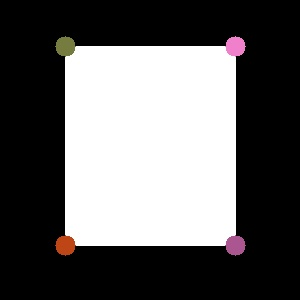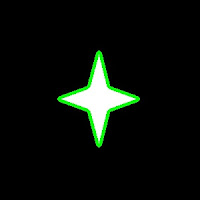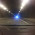## Sunday, June 10, 2012

### Contours - 1 : Getting Started

Hi, this article is a tutorial which try to cover all relevant functions in OpenCV dealing with Structural Analysis and Shape Descriptors, which are mainly related to contours.Contours can be explained simply as a curve joining all the continuous points (along the boundary), having same color or intensity. For example, consider image at left.

Assuming it is a binary image,we can say, its contour is the curve joining the all the boundary white points.

So if we find a contour in a binary image, we are finding the boundaries of objects in an image. That is why, OpenCV doc says, "The contours are a useful tool for shape analysis and object detection and recognition".

Finding Contours:

We start with a simple image as above. First we find the contours.

import numpy as np
import cv2

imgray = cv2.cvtColor(im,cv2.COLOR_BGR2GRAY)
ret,thresh = cv2.threshold(imgray,127,255,0)
contours, hierarchy = cv2.findContours(thresh,cv2.RETR_TREE,cv2.CHAIN_APPROX_SIMPLE)


Points to remember :
1. For better accuracy, use binary images. So before finding contours, apply threshold or canny edge detection.
2. FindContours function modifies the source image, so 'thresh' before and after finding contours are different image. So if you want 'thresh' as such after finding contours, store it to some other variables.
3. In OpenCV, its operation is like finding white object from black background. So remember, object to be found should be white and background in black.
What is structure of resulting contours?

The result "contours" is a Python list, where it contains all objects boundary points as separate lists. So to find number of objects, find length of list "contours", where in this case, it is one. Only one object. So we take it as "cnt".

>>> len(contours)
1
>>> cnt = contours
>>> len(cnt)
244


Here, number of points in cnt is 244. What these points denote? They are the boundary points of the object.

But, does it include all the boundary? Not exactly. The points are selected such that, contours can be drawn as straight line joining these points. So, if object is a horizontal or vertical line, only end points are stored. ie length of cnt = 2. If object is a rectangle, only 4 vertices are stored.Contour points for a rectangle

Thus in our image, there are no direct horizontal or vertical lines. So most of the points will be stored. To visualise it as above, you can draw circles for each value in cnt.

How to draw the contours?

For this, there is a function, cv2.drawContours(). Let's try it:

cv2.drawContours(im,contours,-1,(0,255,0),3)


This draws a 3-pixel wide green outline of the object. If you want to fill the object with a particular color, pass value of -1 to line thickness.

cv2.drawContours(im,contours,-1,(0,255,0),-1)Contours drawn filledContours drawn 3 px wide

Also, the third argument in cv2.drawContours() is also to be noted. Suppose, you want to draw only fourth contour(not here), third argument should be set to 3. If it is -1, all contours are drawn.

Now you want to draw "cnt" only. It can be done as follows:

cv2.drawContours(im,[cnt],0,(255,0,0),-1)


Note the square bracket around "cnt". Third argument set to 0, means only that particular contour is drawn.

Now we end after one more important concept, called Mask.

Mask : What and Why?

Mask can be considered as a binary image where only our desired area is white and all others are blacked out. They are used to isolate a part of image and do operations on that part only without affecting or operating on other parts of the image. This can also be considered as a ROI (Region of Interest) which can have any shape.Consider a scenario, where you are asked to find average colors of each shapes in the image at right. So simply threshold the image to binarize it (please don't ask me if white ball can be detected using thresholding, it is just an example). Find contours in the binary image, then for each contour, create a mask image of that shape. ie, if first ball is cosidered, the region of that ball in mask image will be white, while all other shapes and backgrounds are blacked out. Now if you can find the mean color of that shape only. So for every shapes.

(OK, just for this case, I will do it in this image, not on our original image at the beginning)

First we find the contours as we did before. (Adjust the threshold value to detect all). Now we will see how to do it:

First create a mask image where all elements are zero (ie just a black image) with size same as source, but single channel (ie grayscale).

Then for each contour, we draw it on the mask image filled with white color. Then we find mean using mean() function, taking our mask as operating mask.

for h,cnt in enumerate(contours):Mask Images

See the result at left side.

(All the resulting images are animated to a single image)

I think it is sufficient for now. Keep these three in mind, ie Find Contours, Draw Contours and Mask Image. Now we can find some contour features in next post.

Regards,
ARK

1.Nice tut..

2.Can you please explain how to access the edges of a polygon. I mean x,y values of edges.

1.Sorry, Are you asking about sides of polygon or its vertices? For example, for a triangle ABC, are you asking about vertices (A,B,C) or sides (AB,BC,AC)? Also read the part-2, part-3, part-4 of this series on contours for more info.

2.@abid rahman, i've text and shapes in an image, when i try to get the triangle out- my text is also considered as triangle- i'm using if contours==3, any ideaS?

3.It may be because, when you approximate the contour, it contains only 3 points. Just check it out. You will have to adjust the parameter in cv2.approxPolyDP function to get a good result. (Check reducing its value). I can't tell more without the image you are working with

3.Hi! When I try to draw the contours using cv2.drawContours, I get a TypeError: is not a numpy array.

My function call looks like:

fcontours = cv2.findContours(edges_arr2,
mode = cv2.RETR_TREE,
method = cv2.CHAIN_APPROX_SIMPLE)

then

cv2.drawContours(image = edges_arr,
contours = fcontours,
contourIdx = -1,
color = (0,255,0)
)

where edges_arr2 is a copy of edges_arr, my binary edge image (just a simple rectangle!!!)

Any idea?

Cheers,
Joe

4.cv2.drawContours(im,contours,-1,(0,255,0),3)

is not working for me i am getting this traceback
Traceback (most recent call last):
File "FILENAME.py", line 19, in
cv2.drawContours(im,contours,-1,(0,255,0),3)
TypeError: contours data type = 5 is not supported

what am I doing wrong?

1.Me too get the same error. Problem is with version 2.4.3. I have filed a bug report to OpenCV. Meanwhile, you have to work with version 2.4.2.

5.Hi! Congrats for this amazing blog!
I've got a little problem with 'drawContours' function. It actually does not give any error, but it does not do anything!!
Any hint?

thanks!

6.The code you posted here does not work with 2.4.3 version

cv2.drawContours(im,contours,-1,(0,255,0),3)

By downgrading to 2.4.2 it works :/

1.Me too get the same error. Problem is with version 2.4.3. I have filed a bug report to OpenCV. Meanwhile, you have to work with version 2.4.2.

7.Thanks for these great tutorials.
I get error:
OpenCV Error: Assertion failed (scn == 3 || scn == 4) in unknown function, file ..\..\.

at line:
imgray=cv2.cvtColor(im, cv2.COLOR_BGR2GRAY)

Any ideas???
Thank you!

1.Input image should be 3-channel, ie RGB etc. And it should be loaded correctly. just print im.shape, and check if it is a valid numpy array and shape is (rows,cols,3) where rows and cols are number of rows and columns of your image.

8.Do you have any idea why:
cv2.drawContours(im,contours,-1,(0,255,0),3)

does not produce any output for me? It runs without errors, but does not do anything. Everything else works to that point. Thank you. I am doing this in Spyder.

1.I don't know really. Never came across such a problem. But which version of OpenCV do you use?

2.Hi, I have the same problem, cv2.drawContours(im,contours,-1,(0,255,0),3) does not produce any output, i have installed OpenCV 2.4.2 and Python 2.7.....

3.Hi, I am not sure why it happens. Can you please send me your code and image to my mail : abidrahman2@gmail.com ?

4.Hi, thanks for the great tutorial! I also have the same issue. It seems like none of the drawing functions produce output. I'm also working with opencv 2.4.2, python 2.7 (32 bit) and Eclipse. Any luck with what this is?

5.I think I found a solution for my problem. I needed to use cv2.namedWindow, and then cv2.imshow("windowname", im) for the results of the draw functions to display. Thanks again for the tutorial!

6....and also a cv2.waitkey(0) at the end.

9.How can i see binary images? if i execute: cv2.imshow('im',im) show up only a gray window!

1.Of course you can view a binary image with imshow function. make sure, it has only 1 channel and of datatype= uint8. (you can use img.shape and img.dtype for that)

10.My image have white background and a 3 drawed signs find_contour finds 100 hundred objects instead of only 3! I can change any setting or maybe i could change method to retrieve contours? it's better binary images or canny algorithm as first step (i used binary images)?

1.I can't say it without seeing the image. Anyway, for finding contours, it is best to have a black background and white objects. So invert the image. Also, apply threshold before finding contours,especially if image is jpg format.

11.what does the last line (mean = cv2.mean(im,mask=mask) does?

1.It finds the mean color inside the shape specified by the mask image. ie it calculates the mean of a region in image 'im', where region is specified by the mask image.

2.ok this is clear, then how to display this portion both from the binarized image and from the intensity image? cv2.imshow() does not work. i'm missing something...

3.cv2.imshow() should work. Check my last animated image. It is created by this way only. Once you created the mask image,use imshow() to display it. Also, use bitwise_and() to perform AND operation between mask and intensity image. It will give you the only shape you want. Display that also.

4.thank you. in the end bitwise_and(src,mean,mask = mask) worked perfectly.

5.6.then why compute the mean?

7.Read my statement in italics. My goal was to find the average color of each shape. so cv2.mean() gives you R,G,B values of each shape. In order to show a shape in particular (as in my animated image), use bitwise_and() and imshow().

8.ok, sorry for that! btw, great tutorial :)

12.I copied and pasted your first block of code and set cnt = contours. Why am i getting a different data type?? len(contours) gives 995 and len(cnt) gives 1. Please help thank you!

1.I don't understand why you got like that? You used the same image as above? Then you should get the opposite way, actually.

13.@Abid Rahman Nice. I have a question though. If I have many blobs(some have holes while some do not). It will go through a blobs elimination process. How can I retrieve back the blobs with the holes as well?

1.I am sorry, I didn't get your question.

14.thanks for the tutorial.one question though,the findcontours function returns hierarchy.I checked the documentation and could not comprehend what you could hierarchy for.

1.http://opencvpython.blogspot.com/2013/01/contours-5-hierarchy.html

2.thanks

15.I had the same trouble with this
"cv2.drawContours(im,contours,-1,(0,255,0),3)
TypeError: contours data type = 5 is not supported"
Error on python 2.7.3. with opencv 2.4.3.

So I installed the newest version 2.4.5. of opencv.
Now very thing is working fine :>